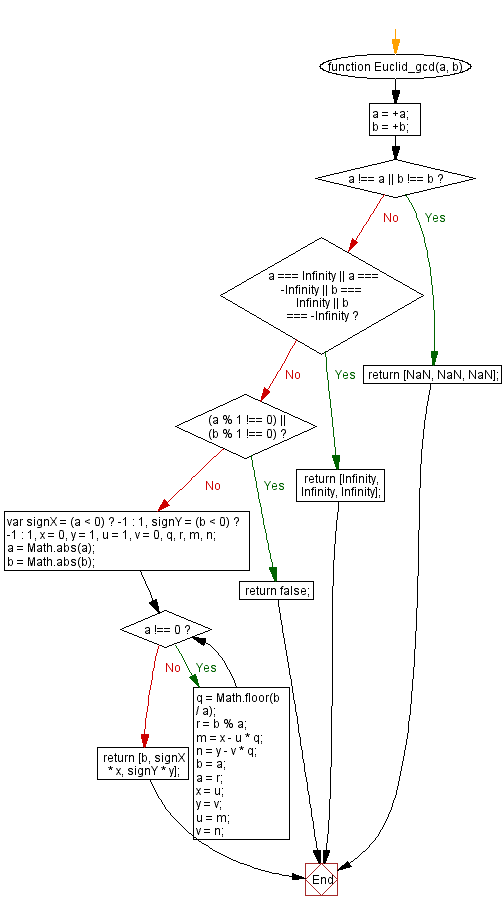# JavaScript: Calculate the extended Euclid Algorithm or extended GCD

## JavaScript Math: Exercise-47 with Solution

Write a JavaScript function to calculate the extended Euclid Algorithm or extended GCD.

In mathematics, the Euclidean algorithm[a], or Euclid's algorithm, is an efficient method for computing the greatest common divisor (GCD) of two numbers, the largest number that divides both of them without leaving a remainder. It is named after the ancient Greek mathematician Euclid, who first described it in Euclid's Elements. It is an example of an algorithm, a step-by-step procedure for performing a calculation according to well-defined rules, and is one of the oldest algorithms in common use. It can be used to reduce fractions to their simplest form, and is a part of many other number-theoretic and cryptographic calculations.

Pictorial Presentation:Sample Solution:-

HTML Code:

``````<!DOCTYPE html>
<html>
<meta charset="utf-8">
<title>JavaScript function to calculate the extended Euclid Algorithm or extended GCD</title>
<body>

</body>
</html>
```
```

JavaScript Code:

``````function Euclid_gcd(a, b) {
a = +a;
b = +b;
if (a !== a || b !== b) {
return [NaN, NaN, NaN];
}

if (a === Infinity || a === -Infinity || b === Infinity || b === -Infinity) {
return [Infinity, Infinity, Infinity];
}
// Checks if a or b are decimals
if ((a % 1 !== 0) || (b % 1 !== 0)) {
return false;
}
var signX = (a < 0) ? -1 : 1,
signY = (b < 0) ? -1 : 1,
x = 0,
y = 1,
u = 1,
v = 0,
q, r, m, n;
a = Math.abs(a);
b = Math.abs(b);

while (a !== 0) {
q = Math.floor(b / a);
r = b % a;
m = x - u * q;
n = y - v * q;
b = a;
a = r;
x = u;
y = v;
u = m;
v = n;
}
return [b, signX * x, signY * y];
}

console.log(Euclid_gcd(17, 4));
```
```

Sample Output:

```[1,1,-4]
```

Flowchart:Live Demo:

See the Pen javascript-math-exercise-47 by w3resource (@w3resource) on CodePen.

Improve this sample solution and post your code through Disqus

What is the difficulty level of this exercise?

Test your Programming skills with w3resource's quiz.

﻿